# 【C++】 C++知识点总结

## 一、C++常用后缀

cpp, .h, cc, cxx, hpp

## 二、头文件

### 1、C++输入输出

• 头文件#include
• 标准输入（standard input）与预定义的 istream 对象 cin 对应
• 标准输出（standard output） 与预定义的 ostream 对象 cout 对应
• 标准出错（standard error）与预定义的的 ostream 对象 cerr 对应

    #include <iostream>
int main(void)
{
int a=0, b=0;
char c=0;
std::cin>>a>>c>>b;
switch(c)
{
case '+':
std::cout<<a<<c<<b<<"="<<a+b<<std::endl; break;
case '-':
std::cout<<a<<c<<b<<"="<<a-b<<std::endl; break;
case '*':
std::cout<<a<<c<<b<<"="<<a*b<<std::endl; break;
case '/':
std::cout<<a<<c<<b<<"="<<a/b<<std::endl; break;
}
return 0;
}


### 2、在C++中使用C的库函数

extern "C"
{
#include <stdlib.h>
#include <string.h>
}


## 三、 指针与动态内存分配

### 1、C

char  *name  =(char*) malloc(100)；
free(name)；


### 2、C++

• 整形数： int *p = new int（10） ； 分配空间并且初始化为 10 释放 delete p
• 整形数组：int *arr = new int ； 分配十个连续整数空间 释放 delete []arr
• 字符型：char *p = new char('a'); 释放 delete p;
• 字符串：char *arr = new char;分配 100 个字符空间 释放 delete []arr;

## 四、命名空间

### 1、作用

• 避免名称冲突；
• 模块化应用程序；
• 匿名的命名空间可避免产生全局静态变量，创建的 “匿名” 命名空间只能在创建它的文件中访问。

### 2、定义

namespace  空间名{
函数，变量， 类型
}

namespace class01
{
std::string name="jack";
int age=19;
int number = 123;
}


### 3、使用空间成员

1、 直接通过空间名::成员名   --标准使用--提倡使用的方法
class01::name   -- ：：所属符号
2、using指示符指引
using namespace calss01; // 把class01空间了里面的内容暴露在当前位置，当文件有变量与命名空间的成员一样时，则后期使用该成员或变量时，程序运行时会报错；但能编译通过
3、 using声明
using class01::number;
// 当文件也有number变量时，则编译的时候就报错，相当于定义了两次该变量。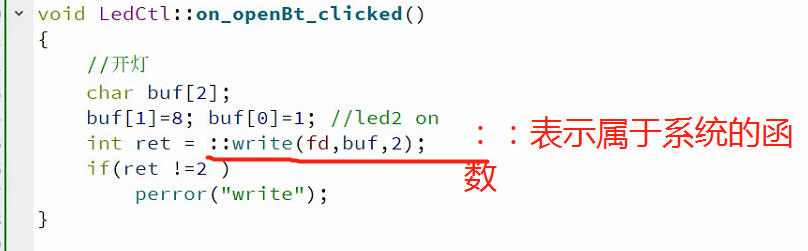### 4、命名空间嵌套

namespace  AAA{
namespace BBB
{
int number=0;
}
}


• AAA::BBB::number;
• using namespace AAA::BBB;   number;
• using AAA::BBB::number;

### 5、匿名空间

static。

namespace {
int data;
}


static 无法修饰自定义类型；static 产生当前 符号只在当前源文件有效，是因为它修改了符号的Bind属性，使之变为局部的；而匿名空间虽然可以产生相同效果，但是符号还是具有外部链接属性。匿名命名空间内的变量与函数，只在当前源文件内有效；不同源文件的匿名命名空间，可以存在同名符合。static需要在每个变量加上

## 六、引用

int a = 10;
int &ra = a; (ra 就是 a 的引用 ，也称 a 的别名)

### 1、引用特点：

• &在此不是求地址运算符，而是起标识作用。
• 类型标识符是指目标变量的类型。
• 声明引用时，必须同时对其进行初始化。
• 引用声明完毕后，相当于目标变量有两个名称即该目标原名称和引用名，且不能再把该引用名作为其他变量名的别名。
• 声明一个引用，不是新定义了一个变量，它只表示该引用名是目标变量名的一个别名，它本身不是一种数据类型，因此引用本身不占存储单元，系统也不给引用分配存储单元，但引用本身是有大小的，一个指针的大小，在64位系统：sizeof(&ra) = 8，32位为4字节,sizeof(ra)=sizeof(a)被引用对象的大小。故：对引用求地址，就是对目标变量求地址。 &ra 与&a 相等。
• 不能建立数组的引用。因为数组是一个由若干个元素所组成的集合，所以无法建立一个

### 2、引用的应用：

1.引用作为参数

2.常引用

3.引用作为函数返回值

• 以引用返回函数值，定义函数时需要在函数名前加&
• 用引用返回一个函数值的最大好处是，在内存中不产生被返回值的副本。
• 不能返回局部变量的引用

## 七、函数重载

int open();
int open(const char* filename);
int open(const char *filename , int flag);
c++中编译程序的是检测函数，通过函数名和参数列表

1. 函数名相同，

3. 如果参数是指针， 或引用，那么const修饰也可以作为重载依据

4. 函数的返回不能作为函数重载的依据

5. 参数是否为默认参数不能作为函数重载的依据

## 八、函数缺省参数（默认参数）

int open(const char *filename, int flag=10)
int open(const char *filename="c++", int flag=10)
int open(const char *filename="c++", int flag) 错误

## 九、类与对象

### 1、类

（1）定义：

 class 类名{
类的特征（属性） 成员变量
类的行为（功能） 成员方法， 函数
}；

class Tdate
{
public:
void Set(int m, int d, int y )
{
month = m ;
day = d ;
year = y ;
}
private:
int month;
int day;
int year;
};


struct和class在C++都能定义类，其区别：

• struct作为数据结构的实现体，它默认的数据访问控制是public的，而class作为对象的实现体，它默认的成员变量访问控制是private的。
• 默认的继承访问权限。struct是public的，class是private的。

（2）类成员权限控制

• public 公有 公有段的成员是提供给外部的接口，在外部可以访问
• protected 保护 保护段成员在该类和它的派生类中可见，在类外不能访问（也就是在外部创建对象时不能访问）
• private 私有 私有段成员仅在类中可见(友元函数或者友元类可以访问)，在类外不能访问（也就是在外部创建对象时不能访问）

（3）类内/外访问

• 类内访问：在类的成员函数中访问成员（没有任何限制）
• 类外访问: 在类的外部通过类的对象访问类的成员

### 2、对象

 class Tdate
{
public:
int num;
void set(int m, int d, int y )
{
month = m ;
day = d ;
year = y ;
}
private:
int month;
int day;
int year;
};

//定义对象
Tdate A;
Tdate *B = new Tdate( );
//对象成员访问
A.set(1,1,1);
A.num = 2;
B->set(1,1,1);
B->num = 2;


## 十、构造和析构函数

### 1、构造函数

（1）如果创建一个类你没有写任何构造函数,则系统会自动生成默认的无参构造函数，函数为空，什么都不做。
（2）只要你写了一个下面的某一种构造函数，系统就不会再自动生成这样一个默认的构造函数，如果希望有一个这样的无参构造函数，则需要自己显示地写出来
（3）参数列表初始化：只有构造函数才有参数列表初始化。若要在类内声明，类外定义构造函数，且使用参数列表初始化参数时，则在类内声明的时候不允许参数列表初始化，只能类外定义的时候进行参数列表初始化
（4）函数默认参数：无论是成员函数还是构造函数，若需要类内声明和类外定义的时候，默认参数值在声明或者定义的时候都可赋值，但声明和定义的参数不能有默认值

#### <1>构造函数定义及重载

class Complex
{
public:
Complex()//构造函数定义
{
m_real = 0.0;
m_imag = 0.0;
}

Complex(int a,int b)//重载构造函数定义
{
m_real = a;
m_imag = b;
}
private :
double m_real;
double m_imag;
};

//创建对象
Complex A；//这个时候不能有（）
Complex A（1，1）；
Complex *A = new Complex( )；//可以有（）也可以没有
Complex *A = new Complex（1，1）；



#### <2>构造函数参数列表初始化

Student(string n="name", int num=1000)
:name(n),number(num){
//name = n;
//number = num;
}

* name、number：是本类里面的成员；
* n、num：是对成员赋的值或者变量；
* 不能在类里面声明的时候用参数列初始化，声明的时候可以加默认值；

class A
{
public:
A(int a,int b){}
}

class B:public A
{
A a;
public:
B(int c,int d ):a(c,d){}
}


• 构造函数是成员函数，必须创建对象后才能调用
• 参数列表初始化是在申请空间的同时就初始化
• 如果成员是const修饰的成员、引用成员、继承时候调用父类构造函数，这几种情况就必须用参数列表初始化。

#### <3>拷贝构造函数

（1）如果没有自定义拷贝构造函数，系统会默认生成一个拷贝构造函数（浅拷贝构造函数，不会拷贝堆空间）

    Student Jack; //构造函数
Student Rose = Jack; //拷贝构造函数
Student Tom (Jack); //拷贝构造函数

后面两种拷贝构造函数不会再次调用构造函数


（2）深拷贝构造函数

class Student
{
public:
Student(int age, const char *n){
this->age = age;
this->name = new char;
strcpy(this->name, n);
cout<<"Student()"<<endl;
}//this指针就是函数调用者
~Student(){
delete []this->name;
cout<<"~Student()"<<endl;
}
//深拷贝构造函数
Student(Student& s)
{
cout<<"Student(Student&)"<<endl;
this->age = s.age;
this->name = new char;
strcpy(this->name, s.name);
//this->name = s.name;
}
private:
int age;
char *name;
};


### 2、析构函数

• 函数名有类一样在函数名前面添加~符号
• 析构函数没有返回值， 也没有参数
• 析构函数在对象销毁的时候自动调用(如果new一个对象，构造函数会自动执行，只有在delete的时候才调用析构函数)

class Complex
{
public:
Complex( )//构造函数定义
{
cout << "complex" << endl;
}
~ Complex( )//析构函数定义
{
cout << "~complex" << endl;
}
};

int main( )
{
Complex a;
Complex *p = new Complex();
delete p;
return 0;
}

complex
complex
~complex
~complex


## 十一、类的内存空间

• 计算一个类的对象占用多少空间用sizeof（类名或对象）
• 类的对象大小是其数据成员（非静态数据段），和虚函数表指针（一个类里最多只能有两个指针，一个是虚函数的指针，一个是虚继承的指针）大小和。普通方法（普通函数）不占用内存，但用virtual修饰的虚函数占用一个指针大小的内存。注：一个指针的大小、内存的对齐方式和编译器有关；64位的话，大小为8；32位的话，大小为4。
• 如果一个类中没有数据成员，也没有虚表那么这个类的大小规定为 1 个字节。

## 十二、类继承

• 新的类从已知的类中得到已有的特征的过程
• 新类叫派生类/子类
• 已知的类叫基类/父类
• 如果直接将派生类的对象赋值给基类对象，派生类自身的成员就会被丢弃，只保留基类继承来的成员。
• 将基类指针指向派生类对象是安全的，因为派生类对象“是”它的基类的对象。但是要注意的是，这个指针只能用来调用基类的成员函数。

class 子类名 ：继承方式 父类
{
子类成员
};


class Base
{
public:
Base() {}
int b;
};

class Child: public Base
{
};


### 1、继承方式

• 公有继承（public）：继承时保持基类中各成员属性不变，并且基类中的private成员被隐藏。派生类的成员只能访问基类中的public/protected成员，而不能访问private成员；派生类的对象只能访问基类中的public成员。
• 保护继承（protected）：继承时基类中各成员属性均变为protected，并且基类中的private成员被隐藏。派生类的成员只能访问基类中的public/protected成员，而不能访问private成员；派生类的对象不能访问基类中的任何的成员。
• 私有继承（private）：继承时基类中各成员属性均变为private，并且基类中private成员被隐藏。派生类的成员也只能访问基类中的public/protected成员，而不能访问private成员；派生类的对象不能访问基类中的任何的成员。

### 2、继承

#### （1）继承构造方法和析构方法的定义和调用

• 当派生类的构造函数为B(){cout << "Afather\n";}时，创建一个派生类默认会调用没有参数的父类构造函数A（）。
• 如果父类构造函数带无默认值参数，派生类构造函数怎么写？
如下：
例子一：

public:
Person(string name, string sex, int age):name(name),sex(sex),age(age) {
cout<<"Person()"<<endl;
}

public:
Student( ):Person("jack","man",19){
cout<<"Student()"<<endl;
}  //==>Person();

class Animal
{
public:
Animal(int w, const char *color, int age){
this->weight = w;
this->age = age;
strcpy(this->color, color);
}
protected:
int weight;
int age;
char color;
};
class Cat:public Animal
{
public:
Cat(int w, const char *color, int age, const char *type):Animal(w, color, age)
{
strcpy(this->type, type);
}
void show()
{
cout << "weight=" << weight << "\nage=" << age << "\ncolor=" << color <<endl;
}
protected:
char type;
};


#### （2）继承后成员函数调用

• 父子类成员函数名相同时，不是重载，这时父类的此函数会别隐藏
• 子类调用成员函数时候会检测子类是否存在，如果存在就调用自己的， 如果不存在就调用父类的（前提是父要有这个函数）
• 如果子类和父同时存在这个函数，一定要调用父类函数，可以用（父类名::函数名( )）调用。

#include <iostream>
using namespace std;

class A{
public:
A(){cout << "Afather\n";}
~A(){cout << "~Afather\n";}
void fun( ){
cout << "father fun\n";
}
};

class B:public A{
public:
B(){cout << "Bchildren\n";}
~B(){cout << "~~Bchildren\n";}
void fun(){
cout << "children fun\n";
}
};

int main( )
{
B x;
x.A::fun( );//调用父类的fun
return 0;
}

Afather
Bchildren
father fun
~~Bchildren
~Afather


### 3、多继承

（1）语法：

class <派生类名>:<继承方式1> <基类名1>,<继承方式2><基类名2>,…
{
<派生类类体>
};


（2）例子1：

class A{
public:
A(){cout<<"A()"<<endl;}
~A(){cout<<"~A()"<<endl;}
protected:
int dataA;
};

class B{
public:
B(){cout<<"B()"<<endl;}
~B(){cout<<"~B()"<<endl;}
protected:
int dataB;
};

class C:public A, public B
{
public:
C(){cout<<"C()"<<endl;}
~C(){cout<<"~C()"<<endl;}
protected:
int dataC;
};


（3）例子2： 如果父类构造函数带参数

继承关系class C:public A, public B

A(int a):dataA(a){cout<<"A()"<<endl;}
B(int b):dataB(b){cout<<"B()"<<endl;}

C(int a, int b, int c):A(a), B(b),dataC(c){cout<<"C()"<<endl;}


（4）多个父类有同名的成员

1. 显示调用对应父类中的成员
c.A::info();
c.B::info();
2. 在子类中添加父类的同名成员
这个时候系统会将父类的同名成员隐藏，调用子类的成员

### 4、虚拟继承

class D :virtual public B{  //虚拟继承
...
};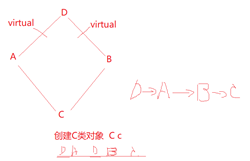class A{
public:
void fun(){}
};

class B:virtual public A{ };
class C:virtual public A{ };

class D:public B,public C{ };
int main(void)
{
D x;
x.fun();//如果不是虚继承，会出现二异性，因为在D类继承了两次A类
return 0;
}


## 十三、虚函数、虚表

virtual void show(){cout<<data<<endl;}

• 如果一个类中包含虚函数， 那么这个类的对象中会包含一个虚表指针vptr
• 虚表指针保存在对象空间的最前面
• 虚表中存储的是类中的虚函数地址
• 对象调用类中虚函数，会查询虚表指针再执行函数
• 一个类里最多只有两个虚表指针（一个是虚函数的指针，一个是虚继承的指针）
• 用virtual修饰的虚函数占用一个指针大小的内存。64位的话，大小为8；32位的话，大小为4。
• 同一个类的不同实例共用同一份虚函数表, 它们都通过一个所谓的虚函数表指针__vfptr(定义为void**类型)指向该虚函数表.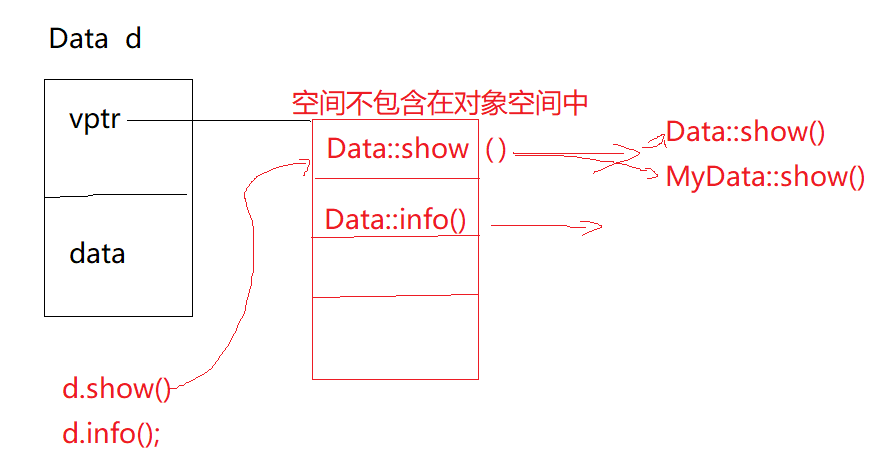#include <iostream>
using namespace std;
class Base
{
public:
Base(){}
virtual ~Base(){}
public:
virtual void show(int a=123){
cout<<"Base::show()"<<a<<endl;
}
};
class Child:public Base
{
public:
Child(){}
~Child(){}
virtual void show(int a=321){
cout<<"Child::show()"<<a<<endl;
}
virtual void info()
{
cout<<"Child::info()"<<endl;
}
};
int main()
{
Child c;
Base *p = &c;
p->show();
return 0;
}

Child::show()123

（1）当show函数不是虚继承时，输出结果为Base::show()123，因为父类的指针只能调用自己的成员，如果有虚继承，则虚表里面父类的show函数的地址会被子类的show函数地址覆盖，被覆盖的前提是：两个函数的名称和参数类型、个数和返回值类型一样。


#include <iostream>
using namespace std;

class Base
{
public:
Base(){}
virtual ~Base(){}
protected:
virtual void show(int a= 0){
cout<<"Base::show()"<<endl;
}
};
class Child:public Base
{
public:
Child(){}
~Child(){}
virtual void show(){
cout<<"Child::show()"<<endl;
}
virtual void info()
{
cout<<"Child::info()"<<endl;
}
};
int main()
{
Child c;
typedef void (*Fun)();
c.show();
Fun f = (Fun)(((long*)(*((long*)(&c)))));
f();
return 0;
}

Child::show()
Base::show()


## 十四、纯虚函数（抽象函数）、抽象类

（1）纯虚函数--虚函数不需要实现直接赋值为0，纯虚函数有时称为抽象函数。

virtual void run()=0;


（2）抽象类

• 如果一个类中包含纯虚函数，那么这个就是抽象类，抽象类是不能创建对象。
• 抽象类可以派生出子类， 如果在子类中没有把父类中的纯虚函数全部实现，那么子类照样是抽象类。

#include <iostream>
#include <windows.h>
#include <time.h>
using namespace  std;
public:
void start();
virtual void run()=0;
protected:
};

void *handle(void *arg)
{
th->run();
}

{
int ret = pthread_create(&id, NULL, handle, (void*)this);
if(ret < 0)
{
cout<<"create fail"<<endl;
}
}

//派生一个线程子类--获取系统时间
public:
virtual void run()
{
while(1)
{
Sleep(1000);
time_t t;
time(&t);
}
}
};

int main()
{
tth.start();
tt.start();
while(1){}
return 0;
}


## 十五、多态、虚析构

### (1)多态

<1>概念
C++中，多态性是指具有不同功能的函数可以用同一个函数名，这样就可以用一个函数名调用不同内容的函数。

（1）静态多态性：在程序编译时系统就能决定调用的是哪个函数，因此又称为编译时的多态性，通过函数的重载实现（运算符重载实际上也是函数重载）；
（2）动态多态性：在程序运行过程中才动态地确定操作所针对的对象，又称为运行时多态性，通过虚函数实现。

<2>动态多态性和虚函数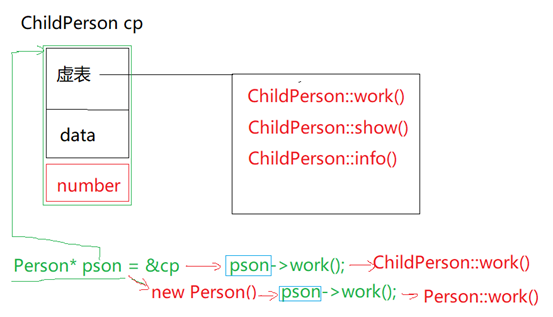#include <iostream>
using namespace std;
class Person{
public:
Person(){}
~Person(){}
virtual void work(){cout<<"Person::work()"<<endl;}
protected:
int data;
};

class ChildPerson: public Person{
public:
ChildPerson(){}
~ChildPerson(){}
virtual void show(){cout<<"ChlidPerson::show()"<<endl;}
virtual void work(){cout<<"ChildPerson::work()"<<endl;}
virtual void info(){}
protected:
int number;
};

class A: public Person{
public:
A(){}
~A(){}
virtual void show(){cout<<"A::show()"<<endl;}
virtual void work(){cout<<"A::work()"<<endl;}
virtual void info(){}
protected:
int num;
};

int main()
{
ChildPerson cp;
A a;
Person* p = &a;
Person* pson = &cp;
pson->work(); //ChildPerson::work();
p->work();//A:work();
return 0;
}


### (2)虚析构

#include<iostream>
using namespace std;
class Base
{
public:
Base(){cout<<"create Base"<<endl;}
virtual ~Base(){cout<<"delete Base"<<endl;}
};
class Der : public Base
{
public:
Der(){cout<<"create Der"<<endl;}
~Der(){cout<<"Delete Der"<<endl;}
};
int main(int argc, char const* argv[])
{
Base *b = new Der;
delete b;
return 0;
}


## 十六、友元

• 友元优点： 可以在函数中直接访问成员数据， 可以适当提高程序效率
• 友元缺点：在函数类的权限失效， 破坏了类的封装性
friend关键声明友元函数，或类。

class Data
{
public:
Data() {}
void setA(int a)
{
this->a = a;
}
protected:
int a;
private:
int b;
//在Data类中声明函数fun为友元函数
friend void fun();
};
void fun()
{
Data data;
//data.a = 120;
data.setA(120);
data.b = 220;
}



class Data
{
public:
Data() {}
void setA(int a)
{
this->a = a;
}
protected:
int a;
private:
int b;
//在Data类中声明函数fun为友元函数
friend void fun();
//声明show为友元函数，show不是成员函数
friend void show()
{
Data data;
data.a = 130;
cout<<data.a<<" "<<data.b<<endl;
}
};
void show(); //在外面声明函数


## 十七、友元类

（1）在 B 类中设置 A 类为友元类。
（2）A::fun 要访问B类中的所有成员， 把A::fun函数声明为B类的友元函数。

（1）例如：在 B 类中设置 A 类为友元类，A类成员函数可以访问B类的protected、private成员，B类不能访问A类，如果要双向访问则要在两个类中声明对方为友元类。友元关系不能被继承。

class B
{
public:
B(){}
friend class A;//在 B 类中声明 A 类为友元类
private:
int bdata;
};
class A
{
public:
A(){}
void showB(B &b)
{
b.bdata = 100;//在 A 类中成员函数使用 B 类的私有数据
}
private:
};


（2）A::fun 要访问B类中的所有成员， 把A::fun函数声明为B类的友元函数

#include <iostream>
using namespace std;
//前向声明----只能用于函数形参， 定义指针， 引用，不能使用类具体成员
class B;
class A{
public:
void fun(B& b);
};
class B{
public:
B(){}
protected:
int mb;
private:
int nb;
friend void A::fun(B& b);//在B类中声明fun为友元函数
};
void A::fun(B& b){
cout<<b.mb<<b.nb<<endl;
}
int main()
{
cout << "Hello World!" << endl;
return 0;
}


## 十八、运算符重载

1. 根据实际应用需求来重载运算符， 重载的时候必须保持不能改变运算符本来的特性
2. 只能重载c++已有的运算符，不能自己新创建运算符

1、那些运算能重载

• 双面运算符  （+，-，*，/， %）
• 关系运算符 （==， !=, <,  >, <=, >=）
• 逻辑运算符 (||,   &&,  !)
• 单目运算符 (*， &， ++， --)
• 位运算符   （|，  &，  ~， ^,  <<, >>）
• 赋值运算符 (=, +=, -=,   .....)
• 空间申请运算符 (new , delete)
• 其他运算符 ((),  ->,  [])

2、那些运算符不能重载

• .(成员访问运算符)
• .*(成员指针访问运算符)
• ::(域运算符)
• sizeof(数据类型长度运算符)
• ?:(条件运算符， 三目运算符)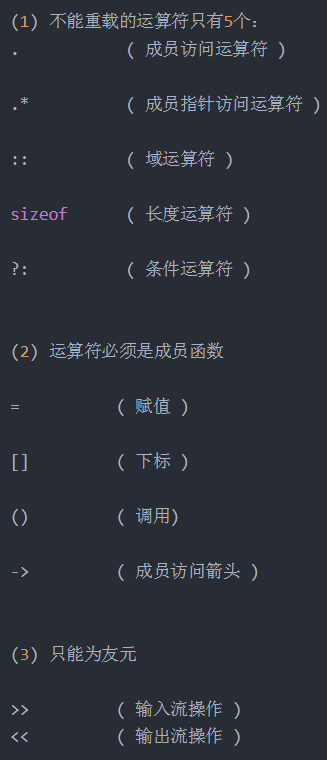3、格式：

返回类型说明符 operator 运算符符号(<参数表>)
{
函数体
}


4、重载方式：
1.重载方式---成员函数重载

Complex C = A+B;    --》A.operator+(B);



2.重载方式--友元重载（普通函数重载）（可以在类里面定义，也可以在类外定义类内声明）

Complex C = A-B;     -->operator-(A, B);



### 1、双目运算符重载

#### （1）+ -重载

#include <iostream>
using namespace std;
class Complex
{
public:
Complex(int r, int i):real(r), image(i) {}
//成员重载实现重载加法+
Complex  operator+ (Complex &B)
{
cout<<"operator"<<endl;
Complex C(0,0);
C.real = this->real +  B.real;
C.image = this->image + B.image;
return C;
}
//成员重载实现 对象加一个整型数
int operator+(const int &a)
{
this->real += a;
this->image += a;
return this->real;
}
private:
int real;
int image;
friend Complex operator- (Complex &A, Complex &B);
};
//友元重载实现重载减法
Complex operator- (Complex &A, Complex &B)
{
Complex C(0,0);
C.real = A.real -  B.real;
C.image = A.image - B.image;
return C;
}
int main()
{
Complex A(2,2);
Complex B(1,1);
Complex C = A+B; //==>A.operator+(B)
int c  =  A+100; //==>A.operator+(100)
Complex D = A-B; //operator-(A, B)
return 0;
}


#### （2）输出、输入， 运算符重载

#include <iostream>
using namespace std;
class Point
{
public:
Point (int x=0, int y=0):x(x),y(y){}
void show()
{
cout<<"("<<x<<","<<y<<")"<<endl;
}
private:
int x, y;
//声明友元函数
friend  ostream& operator<<(ostream &out, Point& p);
friend istream&  operator>>(istream &in, Point& p);
};
//重载输出
ostream&  operator<<(ostream &out, Point& p)
{
out<<"("<<p.x<<","<<p.y<<")"<<endl;
return out;
}
//输入重载
istream&  operator>>(istream &in, Point& p)
{
in>>p.x>>p.y;
return in;
}
int main()
{
Point p(10,20);
p.show();
cout<<p<<endl;   //==> ostream& operator<<(cout, p)
Point A(0,0);
cin>>A;
cout<<A;
return 0;
}


### 3、单目运算符重载

#### （1）++A、A++重载

#include <iostream>
using namespace std;
class Data
{
public:
Data(int d=0):data(d) {}
//重载A++
Data operator++(int)
{
Data old(*this);//保存原先的数捍
this->data += 1;//对原数进行自劍
return old;//返回未加之前的数捍
}
//重载++A
Data& operator++()
{
this->data += 1;//对原数进行自劍
return *this;
}
private:
int data;
friend ostream &operator<<(ostream& out, Data &d);
};
ostream &operator<<(ostream& out, Data &d)
{
out<<d.data<<endl;
return out;
}
int main()
{
Data A;
Data d = A++; //==>A.operator++(int)
cout<<d<<A<<endl;
Data &c = ++A;
cout<<c<<A<<endl;
return 0;
}


#include <iostream>
using namespace std;

class A
{
int data;
public:
A(int d = 0):data(d) {}
void show()
{
cout << this->data << endl;
}
//友元函数重载++A
friend A& operator++ (A &a);
//友元函数重载A++
friend A operator++ (A &b,int);
//友元函数重载<<
friend ostream& operator<< (ostream &out,A &a);
};

//友元函数重载++A
A& operator++ (A &a)
{
a.data += 1;
return a;
}
//友元函数重载A++
A operator++ (A &b,int)
{
A old(b);
b.data += 1;
return old;
}
//友元函数重载<<
ostream& operator<< (ostream &out,A &a)
{
out << a.data;
return  out;
}

int main(int argc,char **argv)
{
A a(5);
A b = ++a;
cout << a << " " << b << endl;

A c(5);
A d = c++;
cout << c << " " << d << endl;

return 0;
}


#### （2）重载中括号[ ]

#include <iostream>
using namespace std;
class Array
{
public:
Array(int n):length(n) {
this->ptr = new int[this->length];
}
~Array(){
delete []this->ptr;
}
//拷贝构造函数---深拷贝（类的成员有指针指向堆空间）
Array(Array& array)
{
this->length = array.length;
this->ptr = new int[this->length];
memcpy(this->ptr, array.ptr , this->length);
}
//重载[]
int& operator[](int i)
{
cout<<i<<endl;
return this->ptr[i];//返回第i个对象
}
private:
int length;
int *ptr;
};

int main()
{
Array mArr(10);
mArr = 100;  //
return 0;
}


### 4、用运算符重载实现数据类型转换

##### （1）转换构造函数

#include <iostream>
using namespace std;
class Complex
{
public:
Complex():real(0),imag(0){cout << "test1\n";}

Complex(double r, double i):real(r),imag(i){cout << "test2\n";}

// 定义转换构造函数
Complex(double r):real(r),imag(0){cout << "test3\n";}

/*
// 拷贝构造函数
Complex(Complex &a){  cout << "test4\n";  }//当此函数存在时，Complex c = 1；Complex c2 = c1 + 3.1;编译时都会报错
*/

void Print(){
cout<<"real = " << real <<" image = "<<imag<<endl;
}

Complex operator+(Complex c){
Complex ret(this->real + c.real, this->imag + c.imag);
return  ret;
}
private:
double real;
double imag;
};
int main()
{
Complex c;
c = 4;  // 调用转换构造函数将1.2转换为Complex类型，此时会调用转换构造函数
c.Print();
Complex c1(2.9, 4.2);
Complex c2 = c1 + 3.1; // 调用转换构造函数将3.1转换为Complex类型
c2.Print();
return 0;
}

test1
test3
real = 4 image = 0
test2
test3
test2
real = 6 image = 4.2


• 1、用转换构造函数可以将一个指定类型的数据转换为类的对象｡但是不能反过来将一个类的对象转换为一个其他类型的数据(例如将一个Complex类对象转换成double类型数据)｡
• 2、如果不想让转换构造函数生效，也就是拒绝其它类型通过转换构造函数转换为本类型，可以在转换构造函数前面加上explicit
##### （2）用运算符重载实现数据类型转换

#include <iostream>
using namespace std;
class Person
{
public:
Person(int age=0):age(age) {}
operator int() //通过运算符重载来实现数据类型转换
{
cout<<"int"<<endl;
return age;
}
operator long() //通过运算符重载来实现数据类型转换
{
cout<<"long"<<endl;
return num;
}
int getAge(){return age;}
private:
int age;
long num;
};
int main()
{
Person Jack(19);
int age = Jack;
int a = Jack.getAge();
long b = Jack;
return 0;
}


• 1、在函数名前面不能指定函数类型,函数没有参数｡
• 2、其返回值的类型是由函数名中指定的类型名来确定的｡
• 3、类型转换函数只能作为成员函数,因为转换的主体是本类的对象，不能作为友元函数或普通函数｡
• 4、从函数形式可以看到,它与运算符重载函数相似,都是用关键字operator开头,只是被重载的是类型名｡double类型经过重载后,除了原有的含义外,还获得新的含义(将一个Complex类对象转换为double类型数据,并指定了转换方法)｡这样,编译系统不仅能识别原有的double型数据,而且还会把Complex类对象作为double型数据处理｡

## 十九、模板函数

1、概念： 如果一个函数实现的功能类似，但是函数参数个数相同类型不同，这样就可以把实在该功能的函数设计为模板函数。

2、格式：

template <typename T>  //T为类型名

函数体
}


3、注意：

• （1）在编译时，根据变量生成实例。
• （2）template   T只对其下面的函数模板有效。如果要定义第二个模板函数时，则要再写template
• （3）typename也可以用class。
• （4）T名字可以随便取。
• （5）当参数不一样时，可以这样定义参数列表template <class T,class Tp>
• （6）参数列表可以带默认类型，template <class T,class Tp = int>。
• （7）模板函数只有在使用（不是运行）的时候才会检测错误。

//设计一个模板函数实现两个对象交换
template <typename T>
void mswap(T &a, T &b)
{
T  c = a;
a = b;
b = c;
}
int main()
{
int a = 10;
int b = 20;
cout<<a<<" "<<b<<endl;
mswap(a, b);
cout<<a<<" "<<b<<endl;
return 0;


//错误，因为不能够确定返回值的类型
template <class T, class Tp>
Tp fun(T &a)
{
return a;
}

//修改，但返回值定死了
template <class T, class Tp = int>
Tp fun(T &a)
{
return a;
}

//调用函数时指定类型
template <class T, class Tp = int>
Tp fun(T &a)
{
return a;
}
int main(void)
{
int a = 2;
double ret = fun<int, double>(a)
}


4、模板函数与函数普通同时存在该如何调用

template <typename T>
void mswap(T &a, T &b)
{
cout<<"template"<<endl;
T  c = a;
a = b;
b = c;
}

//普通函数
void mswap(int &a, int &b)
{
cout<<"std"<<endl;
int c = a;
a = b;
b = c;
}

int a = 10;
int b = 20;
cout<<a<<" "<<b<<endl;
mswap(a, b);//---普通函数
cout<<a<<" "<<b<<endl;

double a = 10;
double b = 20;
cout<<a<<" "<<b<<endl;
mswap(a, b);//---模板函数
cout<<a<<" "<<b<<endl;



## 二十、模板类

### 1、模板类的定义

//设计一个模板类  -模板类的类名 A<T>
//template< class  T ,  class Ty> //A<T, Ty>

template< class T > //A<T>
class A
{
public:
A() {}
protected:
T dataA;
};

int main()
{
A<int> a;//定义模板类对象
return 0;
}


• （1）如果是浮点型或者其他普通类型， 是指针或者是引用 template <double &N,class T=int>
class array{...}
定义对象： array<N,int> a ;这里的 N 必须是全局变量。
• （2）参数列表可以带默认类型，template <class T,class Tp = int> ， 如果是默认类型， 与函数的默认参数类似， 必须是如果从那个一个开始默认， 那么后面的所有模板类型多必须有默认类型。
• （3）如果使用数值为整型（ char， short， int， long） 时候。template <int N,class T=int> class array{...}，这里的N只能是常量不能是变量，例如 array<10,int> a或者const int a = 5; array<a,int>。

### 2、模板类友元重载输出

#include <iostream>

using namespace std;

template< class T >
class MVector{
public:
MVector(){
this->size = 1024;
this->count = 0;
this->ptr = new T[this->size];
}
~MVector(){
delete []this->ptr;
}

//拷贝构造函数
MVector(MVector& mv){
this->size = mv.size;
this->ptr = new T[this->size];
memcpy(this->ptr, mv.ptr, this->size*sizeof(T));
}
//添加数据
void append(const T &data){
this->ptr[this->count] = data;
this->count++;
}
//重载<<追加数据
void operator<<(int data)
{
this->ptr[this->count] = data;
this->count++;
}
#if 1//在类里面定义重载函数
//声明友元重载输出<<
friend ostream& operator<<(ostream& out, MVector &mv)
{
for(int i=0; i<mv.count; i++)
{
out<<mv.ptr[i]<<" ";
}
out<<endl;
return out;
}
#endif
//template<class Ty>
//friend ostream& operator<<(ostream& out, MVector<Ty> &mv);
protected:
int count;
int size;
T* ptr;
};
#if 0 //在类内声明，在类外定义重载函数
//重载输出<<运算符
template< class Ty >
ostream& operator<<(ostream& out, MVector<Ty> &mv)
{
for(int i=0; i<mv.count; i++)
{
out<<mv.ptr[i]<<" ";
}
out<<endl;
return out;
}
#endif
//模板函数在使用（不是运行）该函数的时候才会检查语法

int main()
{
MVector<int> mvs;
mvs.append(100);
mvs<<200;
cout<<mvs;
return 0;
}


### 3、模板类继承

#include <iostream>

using namespace std;

//设计一个模板类A<T>
template< class T >
class A
{
public:
A(T a) {}
protected:
T data;
};

//设计一个子类B 继承模板类A<T> --B类也是模板类
template< class T >
class B: public A<T>
{
public:
B(T a):A<T>(a){}

protected:
int datab;
};

//设计一个子类C 继承模板类A<int> --C类就是一个具体类
class C: public A<int>
{
public:
C():A<int>(10){}
};

int main()
{
A<char> a(10); //模板类创建对象
B<string> b("hello"); //模板类子类创建对象
C c;
return 0;
}


### 4、模板类中的静态成员

#include <iostream>

using namespace std;

template<class T>
class Data
{
public:
Data() {}
void show(T msg)
{
data = msg;
cout<<data<<endl;
}
public:
static  T  data;
};

//类外初始化静态成员
template<class T>
T Data<T>::data ;

int main()
{
//创建一个对象
Data<int> mydata;   //编译的时候会生成一个 T为int的类
mydata.show(100);

Data<string>::data = "hello"; //编译的时候会生成一个T 为string的类
cout<<Data<string>::data<<endl;

Data<string> mystr;
cout<<mystr.data<<endl;
return 0;
}


## 二十一、强制类型转换const_cast、static_cast、reinterpert_cast、dynamic_cast

### 1、const_cast把常量转为变量

#include <iostream>

using namespace std;

int main()
{
const int a = 10;
const int *p = &a;

int *ptr = (int*)(&a);//c语言转换(在c语言可以这样写：int *ptr=&a，只是会警告，一样可以操作，c++不允许)
*ptr = 1;
cout<<a<<endl;
cout<<*ptr<<endl;

int &ra = const_cast<int&>(a);
ra = 2;
cout<<a<<endl;
cout<<ra<<endl;

int *x = const_cast<int*>(p);
*x = 3;
cout<<a<<endl;
cout<<*x<<endl;

return 0;
}

10
1
10
2
10
3



（1）为何要去除const限定

#include <iostream>
using namespace std;

void Printer (int* val,string seperator = "\n")
{
cout << val<< seperator;
}

int main(void)
{
const int consatant = 20;
//Printer(consatant);//Error: invalid conversion from 'int' to 'int*'
Printer(const_cast<int *>(&consatant));

return 0;
}


### 2、static_cast静态转化

static_cast < type-id > ( expression )该运算符把expression转换为type-id类型，但没有运行时类型检查来保证转换的安全性。它主要有如下几种用法：

• ①用于类层次结构中基类（父类）和派生类（子类）之间指针或引用的转换，不允许不相关的类进行转换。
进行上行转换（把派生类的指针或引用转换成基类表示）是安全的；
进行下行转换（把基类指针或引用转换成派生类表示）时，由于没有动态类型检查，所以是不安全的。
• ②用于基本数据类型之间的转换，如把int转换成char，把int转换成enum。这种转换的安全性也要开发人员来保证。
• ③把空指针转换成目标类型的空指针。
• ④把任何类型的表达式转换成void类型。

#include <iostream>
using namespace std;
int main()
{
char a = 'a';
int b = (int)a;
double g = static_cast<int>(a);

//为什么不能转换普通类型指针，却能转换对象指针和void指针(规定的)
void *pp;
double *pp1 = static_cast <double*>(pp);
int *xx;
void *xx1 = static_cast <void*>(xx);
//double *xx2 = static_cast <double*>(xx);//错误写法
return 0;
}


### 3、reinterpret_cast强制类型转换符

reinterpret_cast (expression)
type-id 必须是一个指针、引用、算术类型、函数指针或者成员指针。它可以把一个指针转换成一个整数，也可以把一个整数转换成一个指针（先把一个指针转换成一个整数，再把该整数转换成原类型的指针，还可以得到原先的指针值）。

• reinterpret_cast可以转换任意一个32bit整数，包括所有的指针和整数。可以把任何整数转成指针，也可以把任何指针转成整数，以及把指针转化为任意类型的指针。但不能将非32bit的实例转成指针。总之，只要是32bit的东东，怎么转都行！
• 因为任何指针可以被转换到void，而void可以被向后转换到任何指针（对于static_cast<> 和 reinterpret_cast<>转换都可以这样做），如果没有小心处理的话错误可能发生。

#include <iostream>
using namespace std;
class A {
public:
int m_a;
};
class B {
public:
int m_b;
};
class C : public A, public B {};
int main()
{
int n= 1231651 ;
double *d;
cout << d << endl;
d=reinterpret_cast<double*> (&n);
//为什么d和n的地址一样但为什么地址里面的值不一样？
//是因为double类型数据存储的方式不一样，用*d访问时，
//系统会以读取double类型数据来读取。
cout << d << "  " << &n << endl;
cout << *d << "  " << n << endl;
cout << "---------------------------\n";

//将一个32位的整数转换成一个指针
char *n_p = reinterpret_cast<char*>(10);

//reinterpret_cast和static_cast的主要区别在于多继承
C c;
printf("%p, %p, %p", &c, reinterpret_cast<B*>(&c), static_cast <B*>(&c));//前两个的输出值是相同的，最后一个则会在原基础上偏移4个字节，这是因为static_cast计算了父子类指针转换的偏移量，并将之转换到正确的地址（c里面有m_a,m_b，转换为B*指针后指到m_b处），而reinterpret_cast却不会做这一层转换。

return 0;
}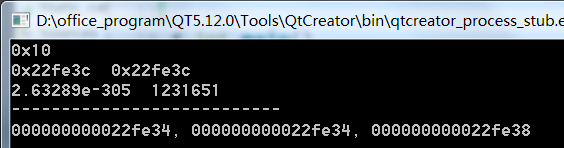//强制类型转换//编写一个程序跳转到地址0x12345678运行
typedef void(*Fun)(void);//定义一个函数指针数据类型
Fun fun = reinterpret_cast<Fun>( 0x12345678 );
fun();


### 4、dynamic_cast类转换

dynamic_cast < type-id > ( expression )

① dynamic_cast是动态转换，只有在基类指针转换为子类指针时才有意义。
② dynamic_cast<>需要类成为多态，即包括“虚”函数，并因此而不能成为void*。
③ static_cast和dynamic_cast可以执行指针到指针的转换，或实例本身到实例本身的转换，但不能在实例和指针之间转换。static_cast只能提供编译时的类型安全，而dynamic_cast可以提供运行时类型安全。

//dynamic---用于继承过程中把父类指针转换为子类指针
#include <iostream>

using namespace std;
class A
{
public:
A() {}
virtual ~A(){}
};
class B:public A
{
public:
B() {}
~B(){}
};
//调用
int main()
{
A *p = new B(); //用户子类指针初始化父类指针
A *a = new A();//创建一个A类对象
//以下两句必须在基类有虚析构的情况下才正确，否则编译的时候报错
B *bptr = dynamic_cast<B*>(a);//把新的父类指针赋值子类指针（nullptr）
B *bptr1 = dynamic_cast<B*>(p);//p是A类型指向B对象空间，把p转回B类
if(bptr1 == nullptr)//为nullptr时转换不成功
{
cout<<"fail"<<endl;
}
else {
cout<<"success"<<endl;
}
return 0;
}


## 二十二、异常捕捉和处理

### （1）异常抛出和捕捉语句

//1.抛出异常
throw  异常对象

//2.异常捕捉
try{
可能会发生异常的代码
}catch(异常对象){
异常处理代码
}

• throw子句：throw 子句用于抛出异常，被抛出的异常可以是C++的内置类型（例如： throw int(1);），也可以是自定义类型。
• try区段：这个区段中包含了可能发生异常的代码，在发生了异常之后，需要通过throw抛出。
• catch子句：每个catch子句都代表着一种异常的处理。catch子句用于处理特定类型的异常。catch块的参数推荐采用地址传递而不是值传递，不仅可以提高效率，还可以利用对象的多态性。

### （2）异常的处理规则

• throw抛出的异常类型与catch抓取的异常类型要一致；
• throw抛出的异常类型可以是子类对象，catch可以是父类对象；
• catch块的参数推荐采用地址传递而不是值传递，不仅可以提高效率，还可以利用对象的多态性。另外，派生类的异常捕获要放到父类异常扑获的前面，否则，派生类的异常无法被扑获；
• 如果使用catch参数中，使用基类捕获派生类对象，一定要使用传递引用的方式，例如catch (exception &e)；
• 异常是通过抛出对象而引发的，该对象的类型决定了应该激活哪个处理代码；
• 被选中的处理代码是调用链中与该对象类型匹配且离抛出异常位置最近的那一个；
• 在try的语句块内声明的变量在外部是不可以访问的，即使是在catch子句内也不可以访问；
• 栈展开会沿着嵌套函数的调用链不断查找，直到找到了已抛出的异常匹配的catch子句。如果抛出的异常一直没有函数捕获(catch)，则会一直上传到c++运行系统那里，导致整个程序的终止。

### （3）实例

• 实例1：抛出自定义类型异常
class Data
{
public:
Data() {}
};

void fun(int n)
{
if(n==0)
throw 0;//抛异常 int异常
if(n==1)
throw "error"; //抛字符串异常
if(n==2)
{
Data data;
throw data;
}
if(n>3)
{
throw  1.0;
}
}

int main()
{
try {
fun(6);//当异常发生fun里面，fun以下代码就不会再执行，调到catch处执行异常处理代码，后继续执行catch以外的代码。当throw抛出异常后，没有catch捕捉，则整个程序会退出，不会执行整个程序的以下代码
cout<<"*************"<<endl;
}catch (int  i) {
cout<<i<<endl;
}catch (const char *ptr)
{
cout<<ptr<<endl;
}catch(Data &d)
{
cout<<"data"<<endl;
}catch(...)//抓取 前面异常以外的所有其他异常
{
cout<<"all"<<endl;
}
return 0;
}

• 实例2：标准出错类抛出和捕捉异常
#include <iostream>
using namespace std;

int main()
{
try {
char* p = new char[0x7fffffff];  //抛出异常
}
catch (exception &e){
cout << e.what() << endl;   //捕获异常，然后程序结束
}
return 0;
}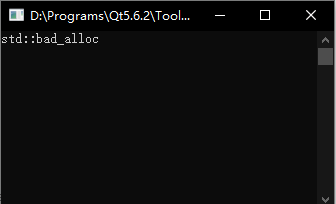• 实例3：继承标准出错类的派生类的异常抛出和捕捉
#include <iostream>
#include <exception>
#include <sys/types.h>
#include <sys/stat.h>
#include <fcntl.h>
using namespace std;
class FileException :public exception
{
public:
FileException(string msg) {
this->exStr = msg;
}
virtual const char*what()  const noexcept//声明这个函数不能再抛异常
{
return this->exStr.c_str();
}
protected:
string exStr;
};

void fun()
{
int fd = ::open("./open.txt",O_RDWR);

if(fd<0)
{
FileException openFail("open fail"); //创建异常对象
throw  openFail;//抛异常
}
}

int main( )
{
try {
fun();
} catch (exception &e) {//一般需要使用引用
cout<<e.what()<<endl;
}
cout<<"end"<<endl;
return 0;
}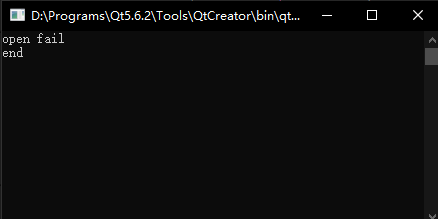98标准写法

~FileException()throw(){}//必须要
virtual const char*what()  const throw()//声明这个函数不能再抛异常
{
return this->exStr.c_str();
}
//编译
g++ main.cpp


2011标准写法

~FileException()noexcept{}//必须要
virtual const char*what()  const noexcept//声明这个函数不能再抛异常
{
return this->exStr.c_str();
}
//编译
g++ main.cpp -std=c++11   指定用c++11标准编译


### （4）总结

1. 使用异常处理的优点：

• 传统错误处理技术，检查到一个错误，只会返回退出码或者终止程序等等，我们只知道有错误，但不能更清楚知道是哪种错误。使用异常，把错误和处理分开来，由库函数抛出异常，由调用者捕获这个异常，调用者就可以知道程序函数库调用出现的错误是什么错误，并去处理，而是否终止程序就把握在调用者手里了。

2. 使用异常的缺点：

• 如果使用异常，光凭查看代码是很难评估程序的控制流：函数返回点可能在你意料之外，这就导致了代码管理和调试的困难。启动异常使得生成的二进制文件体积变大，延长了编译时间，还可能会增加地址空间的压力。
• C++没有垃圾回收机制，资源需要自己管理。有了异常非常容易导致内存泄漏、死锁等异常安全问题。 这个需要使用RAII来处理资源的管理问题。学习成本较高。
• C++标准库的异常体系定义得不好，导致大家各自定义各自的异常体系，非常的混乱。

3. 什么时候使用异常？

• 建议：除非已有的项目或底层库中使用了异常，要不然尽量不要使用异常，虽然提供了方便，但是开销也大。

4. 程序所有的异常都可以catch到吗？

• 并非如此，只有发生异常，并且又抛出异常的情况才能被catch到。例如，数组下标访问越界的情况，系统是不会自身抛出异常的，所以我们无论怎么catch都是无效的；在这种情况，我们需要自定义抛出类型，判断数组下标是否越界，然后再根据自身需要throw自定义异常对象，这样才可以catch到异常，并进行进一步处理。

## 二十三、STL标准模板库

• vector---顺序存储---顺序表 （访问遍历查询）
• list ------链式存储 ----链表  （适合数据长度不确定， 经常改变）
• map ----键值对存储 （key：value）       （适合数据成对存储）
• set ------容器-----------------------（存储数据是唯一的）

### 1、vector（顺序表）

#include <iostream>
#include <vector>
using namespace std;

int main()
{
//创建vector对象
//大小变化:1024  2048  4096（开始会以2倍数增加，后面慢慢以1/3、1/5等的形式增加）
vector<string> names;
//赋值，3个jack
names.assign(3,"Jack"); //Jack, Jack, Jack

//插入数据
//创建一个迭代器
vector<string>::iterator it = names.begin();
//insert之后迭代器it已经改变，返回值为插入值的位置
it = names.insert(++it, "Rose"); //结果：Jack Rose Jack Jack
it = names.insert(++it,"Jim");//结果：Jack Rose Jim Jack Jack
it = names.insert(++it, "lcg"); //结果：Jack Rose Jim lcg Jack Jack
it = names.insert(names.end(), "Jack"); //结果：Jack Rose Jim lcg Jack Jack Jack

//查询数据/删除数据---迭代器遍历使用vector， list， map， set
//names.end()为顺序表最后一个元素的下一个地址
for(it = names.begin(); it != names.end(); ++it)
{
if(*it == "Jack")
{
cout<<*it<<" ";

//擦除，返回擦出元素的下一个位置
//例如a b c，删除b后，返回迭代器指向c
it=names.erase(it);
--it;
}
}
cout<<endl;

//遍历---顺序表
for(int i=0; i<names.size(); i++)
{
cout<<names[i]<<" ";
}
cout<<endl;

return 0;
}

posted @ 2021-04-11 09:42  李春港  阅读(376)  评论(5编辑  收藏